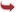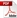International Journal of Scientific and Research Publications

#### IJSRP, Volume 6, Issue 9, September 2016 Edition [ISSN 2250-3153]Midpoints between the Planets and the Gravitational Force at these Midpoints
Eric Sullivan
Abstract: The midpoints determined in this paper were calculated by the use of the distance equation. The gravitation at these midpoints were determined by a modified gravitation equation. The values from both NASA and “Equations for Planetary Ellipses” were used for each midpoints. The NASA’s distances and values were considered as the accepted values and the values determined in “Equations for Planetary Ellipses” are the measured values.
[VIEW FULL PAPER][DOWNLOAD]

Reference this Research Paper (copy & paste below code):

Eric Sullivan (2018); Midpoints between the Planets and the Gravitational Force at these Midpoints; Int J Sci Res Publ 6(9) (ISSN: 2250-3153). http://www.ijsrp.org/research-paper-0916.php?rp=P575797